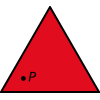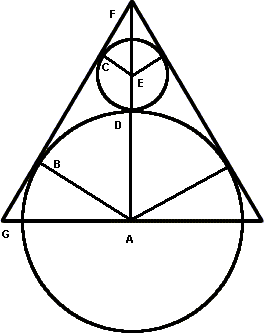#### You may also like### Fitting In

The largest square which fits into a circle is ABCD and EFGH is a square with G and H on the line CD and E and F on the circumference of the circle. Show that AB = 5EF. Similarly the largest equilateral triangle which fits into a circle is LMN and PQR is an equilateral triangle with P and Q on the line LM and R on the circumference of the circle. Show that LM = 3PQ### Triangle Midpoints

You are only given the three midpoints of the sides of a triangle. How can you construct the original triangle?### Three Way Split

Take any point P inside an equilateral triangle. Draw PA, PB and PC from P perpendicular to the sides of the triangle where A, B and C are points on the sides. Prove that PA + PB + PC is a constant.

# Tricircle

##### Age 14 to 16Challenge Level

This solution comes from Soh Yong Sheng (Raffles Institution, Singapore).

First he added a few lines and letters to the diagram:Since the circles are tangent to the triangles, we know that CE is perpendicular to GF, and AB is perpendicular to GF. [A line touching a circle (tangent) is always perpendicular to the radius which meets it.] We also see by symmetry that FA is perpendicular to AG.

The line FA bisects the original equilateral triangle, so we know that FGA=60 °, and GFA=30 °. We can now see that in triangle FCE, CEF=60 °, and in triangle ABF, BAF=60 °. So all three of these triangles have angles 30 °, 60 °, 90 °.Soh Yong Sheng then used trigonometry on these triangles. For those of you who haven't met trigonometry, notice that a triangle with these angles is half of an equilateral triangle, and so the shortest side is half the length of the longest. This tells us that EF = 2CE (from triangle FCE), and FA = 2BA (from triangle ABF).

We know that CE is 1 unit, so EF = 2 units. ED is also a radius of the small circle, so the total length of DF is 3 units.
Now in triangle ABF, FA is twice BA, but BA and DA are both radii, and so must be the same. This means that DF is half of FA, so FA is 6 units, and DA is 3 units. This is the radius of the larger circle.

We also received solutions from Alex and Prateek ( Riccarton High School, Christchurch, New Zealand), and Ramani (Australia). Well done to everyone.